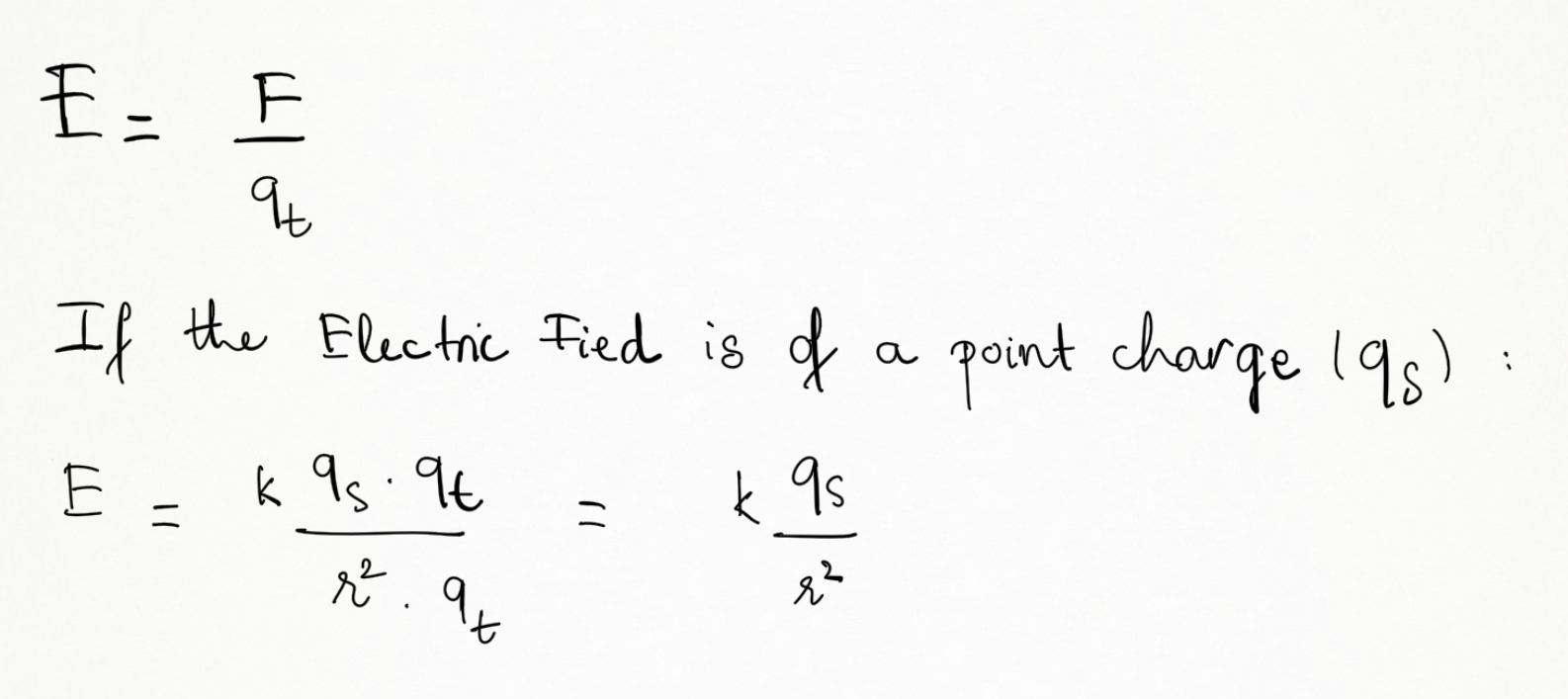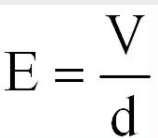Cyber Sale - Only 3 Days Remaining: ALL MCAT Products & Admissions Services

MCAT Content / Electrostatics / Electric Field E

### Electric Field E

Topic: Electrostatics

A region around a charged particle in which an electrostatic force would be exerted on other charged particles is called an electric field. Charged particles accelerate in electric fields.

The electric field (E) at any point is defined as the amount of electrostatic force (F) that would be exerted on a charge of (+1C). To find the electric field, we take the force exerted on the test charge and divide by the magnitude of that test charge:The electric field (E) can also be worked out by dividing the voltage of the electric field by the distance (d) in metres. This gives us the electric field strength in V/m-1.Near a point charge, the strength of an electric field can be calculated as E = kq/r2, where k is a constant and r is the distance from the point charge.

Electric field lines help us visualize the direction and magnitude of electric fields. The properties of electric field lines can be summarized by these rules:

1. The direction of the electric field is tangent to the field line at any point in space.

2. The strength of the field is proportional to the closeness of the lines.

3. Electric field lines can never cross.

4. Electric field lines originate on positive charges and terminate on negative charges.

The electric fields of multiple sources on a test charge can be found by superimposing or adding individual effects.

The electric field due to a continuous charge distribution is found by treating charge elements as point charges and then summing, via integration, the electric field vectors produced by all the charge elements to find the net vector.

Practice Questions

Electric Field Treatments and electroporation

MCAT Official Prep (AAMC)

Physics Question Pack Passage 1 Question 3

Physics Question Pack Passage 4 Question 24

Physics Question Pack Passage 4 Question 27

Physics Question Pack Passage 5 Question 32

Physics Question Pack Passage 5 Question 34

Physics Question Pack Question 78

Section Bank C/P Section Passage 1 Question 1

Sample Test C/P Section Passage 2 Question 5

Practice Exam 3 C/P Section Passage 4 Question 20

Practice Exam 4 C/P Section Passage 3 Question 14

Key Points

• The strength of any point in an electric field can be found by dividing the force exerted on the test charge by the magnitude of that test charge.

• Electric field lines help us visualize the direction and magnitude of electric fields, originate on positive charges and terminate on negative charges.

• The effect of electric fields can be found by superimposing its E vectors.

• The electric field (E) can also be worked out by dividing the voltage of the electric field by the distance (d) in metres. E= V/d.

Key Terms

Test charge: is a charge with a magnitude so small that placing it at a point has a negligible effect on the field around the point.

Coulomb’s constant is ke ≈ 9×109 N⋅m2⋅C−2

Electric field: a region around a charged particle or object within which a force would be exerted on other charged particles or objects

Electrostatic force: the attractive or repulsive force between two electrically charged objects

Field line: a graphical visual aid for visualizing vector fields. It consists of a directed line which is tangent to the field vector at each point along its length

Billing Information